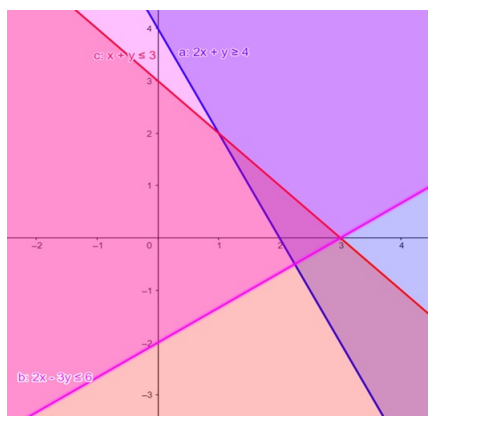# Solve the given inequalities

Question:

Solve the given inequalities $2 x+y \geq 4, x+y \leq 3,2 x-3 y \leq 6$ graphically in two-dimensional plane.

Solution:

The graphical representation of $2 x+y \geq 4, x+y \leq 3,2 x-3 y \leq 6$ is given by common region in the figure below.

$2 x+y \geq 4 \ldots \ldots$ (1)

$x+y \leq 3 \ldots \ldots$ (2)

$2 x-3 y \leq 6 \ldots \ldots$ (3)

Inequality (1) represents the region above line $2 x+y=4$ (including the line $2 x+y=4$ ).

Inequality (2) represents the region below line $x+y=3$ (including the line $x+y=3$ ).

Inequality (3) represents the region above line $2 x-3 y=6$ (including the line $2 x-3 y=6$ ).

Therefore,every point in the common shaded region including the points on the respective lines represents the solution for the given inequalities.

This can be represented as follows,